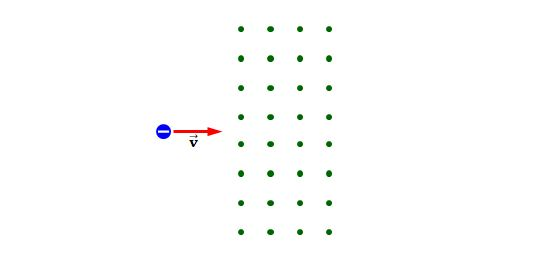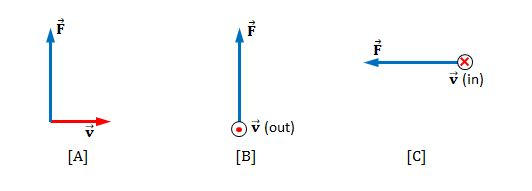Electricity and Magnetism

# Lorentz force law (magnetic fields)A negatively charged particle moving horizontally enters a region where a uniform magnetic field is directed perpendicular to the particles's velocity (into the screen), as shown in the above figure. After the particle enters the field, does it

(a) deflect upward, with its speed remaining constant;
(b) continue to move in the horizontal direction with constant velocity;
(c) move in a circular orbit and become trapped by the field;
(d) deflect downward, moving in a semicircular path with constant speed, and exit the field moving to the left; or
(e) deflect out of the plane of the paper?

Choose coordinates $x$ and $y$ on a horizontal 2-d graphene sheet. We will inject electrons into the sheet from the origin $O$ such that they enter the upper half-plane ($y>0$) but the direction is otherwise totally random. The speed of electrons in graphene is $v_F$, and the mass of each electron is $m$.

We now put an electron detector along the $x$-axis ($y=0$) and apply an external magnetic field $B_0$ perpendicular to the plane (parallel with the $z$-axis). At what value of $x$ in meters will the detector receive the greatest signal?

Assume that every electron that goes to the lower half plane ($y<0$) will disappear (for example, the lower half plane is made of a special material which absorbs electrons).

Details and assumptions

To make the problem numerically simpler, use the following values for your calculation:

• $v_F=1~\mbox{m/s}$

• $m=1~\mbox{kg}$

• $B_0=1~\mbox{T}$

• The electron charge is $e=1~\mbox{C}$.

When a charged particle is traveling through a uniform magnetic field, which of the following statements are true for that magnetic field?

(a) It increases the kinetic energy of the particle.
(b) It exerts a force on the particle along the direction of its motion.
(c) It exerts a force on the particle that is parallel to the field.
(d) It doesn't change the magnitude of the momentum of the particle.
(e) It exerts a force that is perpendicular to the direction of motion.

Consider an electron near the Earth's equator. In which direction does it tend to deflect if its velocity is (a) directed downward and (b) directed westward?What are the directions of the magnetic fields acting on the positively charged particle moving in the various situations shown in the above figure, if the directions of the magnetic forces acting on it are as indicated?

×